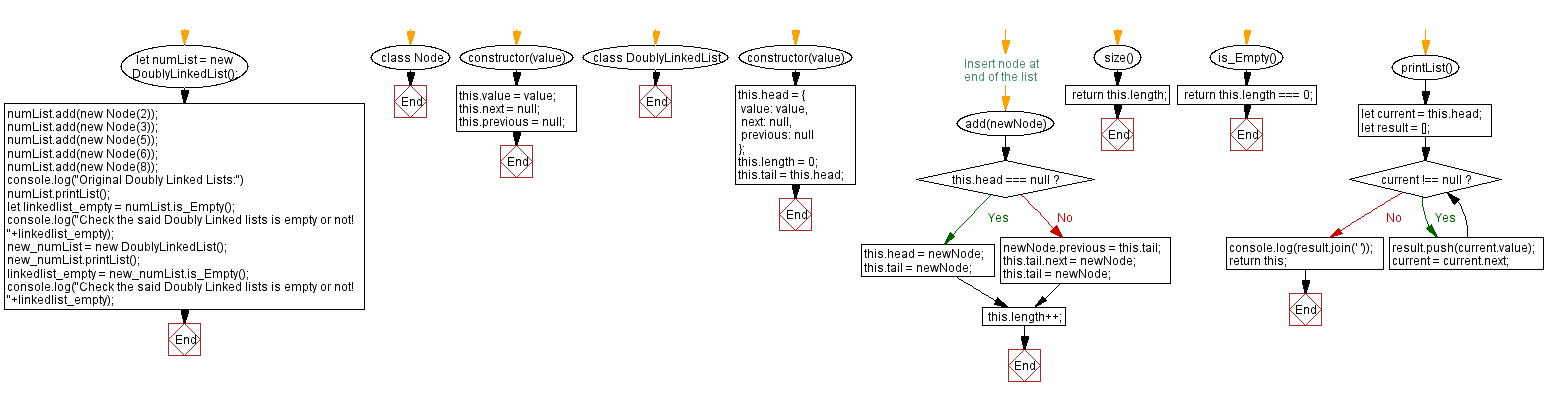# JavaScript Exercises: Check whether a Doubly Linked Lists is empty or not

## JavaScript Data Structures: Exercise-3 with Solution

Write a JavaScript program to check whether a Doubly Linked Lists is empty or not. Return true otherwise false.

Sample Solution:

JavaScript Code:

``````class Node {
constructor(value) {
this.value = value;
this.next = null;
this.previous = null;
}
}

constructor(value) {
value: value,
next: null,
previous: null
};
this.length = 0;
}

// Insert node at end of the list

this.tail = newNode;
}
else
{
newNode.previous = this.tail;
this.tail.next = newNode;
this.tail = newNode;
}

this.length++;
}

size() {
return this.length;
}

is_Empty(){
return this.length === 0;
};

printList() {
let result = [];
while (current !== null) {
result.push(current.value);
current = current.next;
}
console.log(result.join(' '));
return this;
}
}

numList.printList();
new_numList.printList();
```
```

Sample Output:

```Original Doubly Linked Lists:
2 3 5 6 8
Check the said Doubly Linked lists is empty or not! false

Check the said Doubly Linked lists is empty or not! true
```

Flowchart:Live Demo:

See the Pen javascript-doubly-linked-list-exercise-3 by w3resource (@w3resource) on CodePen.

Improve this sample solution and post your code through Disqus

Linked List Previous: Count number of nodes in a Doubly Linked Lists.

What is the difficulty level of this exercise?

Test your Programming skills with w3resource's quiz.

﻿

## JavaScript: Tips of the Day

The unary operator

```let num = 10;

const increaseNumber = () => num++;
const increasePassedNumber = number => number++;

const num1 = increaseNumber();
const num2 = increasePassedNumber(num1);

console.log(num1);
console.log(num2);
```

The unary operator ++ first returns the value of the operand, then increments the value of the operand. The value of num1 is 10, since the increaseNumber function first returns the value of num, which is 10, and only increments the value of num afterwards.
num2 is 10, since we passed num1 to the increasePassedNumber. number is equal to 10(the value of num1. Again, the unary operator ++ first returns the value of the operand, then increments the value of the operand. The value of number is 10, so num2 is equal to 10.

Ref: https://bit.ly/323Y0P6

We are closing our Disqus commenting system for some maintenanace issues. You may write to us at reach[at]yahoo[dot]com or visit us at Facebook